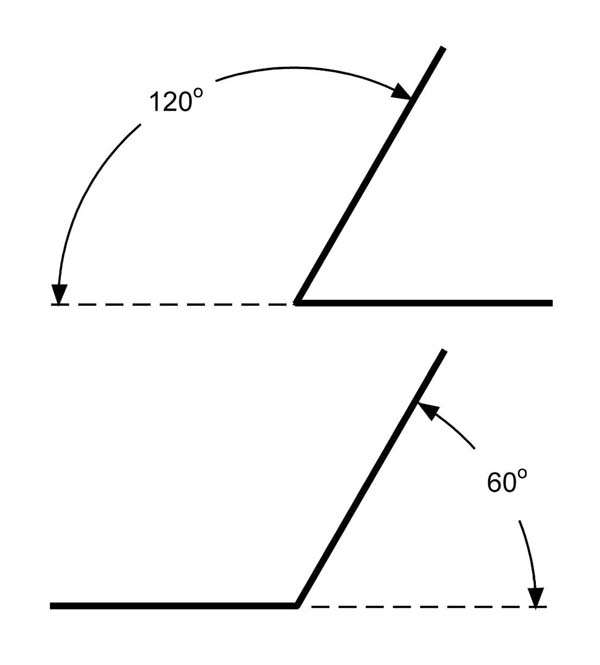Tri/Hex AnglesTri Angles make equilateral triangles, or a 120° transition.
Hex Angles make hexagons, or a 60° transition.

• 12 sizes: 1" to 12" in 1 inch increments.
• Labeled: size is stamped into the metal for easy identification.
• Oversized: sized up 1/8" to include a layer of fiber.
• Stainless steel: long life, stable in the kiln.
• Light guage material: easily make other shapes or true up old ones.

Tri (120o) Equal Leg Angles

 SC_A120_1 #1 equal leg angle \$ 2.00
 SC_A120_2 #2 equal leg angle \$ 3.20
 SC_A120_3 #3 equal leg angle \$ 4.40
 SC_A120_4 #4 equal leg angle \$ 5.60
 SC_A120_5 #5 equal leg angle \$ 6.80
 SC_A120_6 #6 equal leg angle \$ 8.00
 SC_A120_7 #7 equal leg angle \$ 9.20
 SC_A120_8 #8 equal leg angle \$ 10.40
 SC_A120_9 #9 equal leg angle \$ 11.60
 SC_A120_10 #10 equal leg angle \$ 12.80
 SC_A120_11 #11 equal leg angle \$ 14.00
 SC_A120_12 #12 equal leg angle \$ 15.20

Hex (60o) Equal Leg Angles

 SC_A60_1_E #1 equal leg angle \$ 2.00
 SC_A60_2_E #2 equal leg angle \$ 3.20
 SC_A60_3_E #3 equal leg angle \$ 4.40
 SC_A60_4_E #4 equal leg angle \$ 5.60
 SC_A60_5_E #5 equal leg angle \$ 6.80
 SC_A60_6_E #6 equal leg angle \$ 8.00
 SC_A60_7_E #7 equal leg angle \$ 9.20
 SC_A60_8_E #8 equal leg angle \$ 10.40
 SC_A60_9_E #9 equal leg angle \$ 11.60
 SC_A60_10_E #10 equal leg angle \$ 12.80
 SC_A60_11_E #11 equal leg angle \$ 14.00
 SC_A60_12_E #12 equal leg angle \$ 15.20